# Introduction to Programming Using Python v1.0

Page:    1 / 3
Exam contains 43 questions

DRAG DROP -
You are writing a Python program. The program collects customer data and stores it in a database.
The program handles a wide variety of data.
You need to ensure that the program handles the data correctly so that it can be stored in the database correctly.
Match the data type to the code segment. To answer, drag the appropriate data type from the column on the left to its code segment on the right. Each data type may be used once, more than once, or not at all.
Select and Place: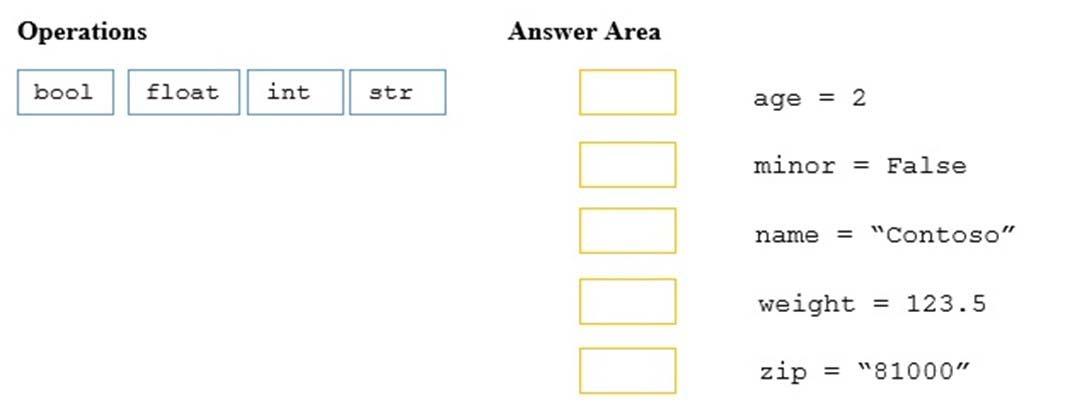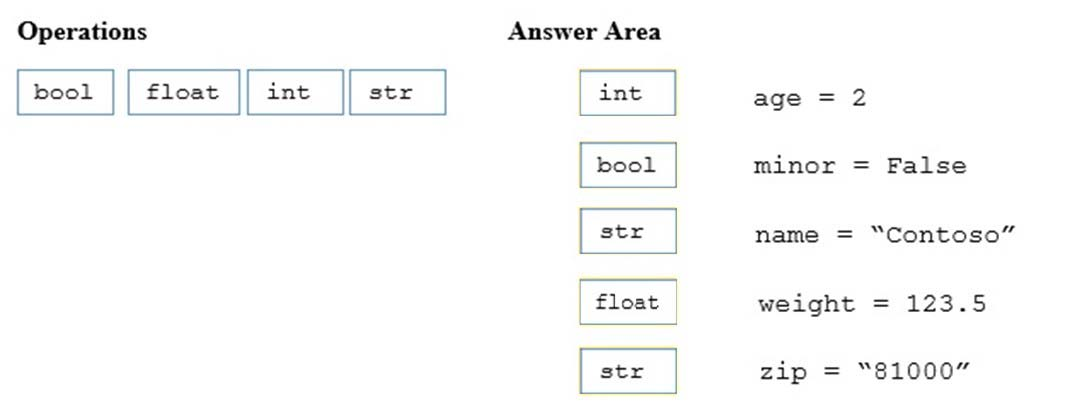References: https://www.w3resource.com/python/python-data-type.php

You are creating a Python program that shows a congratulation message to employees on their service anniversary.
You need to calculate the number of years of service and print a congratulatory message.
You have written the following code. Line numbers are included for reference only.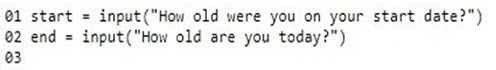You need to complete the program.
Which code should you use at line 03?

• A. print("Congratulations on" + (int(end)-int(start)) + "years of service!")
• B. print("Congratulations on" + str(int(end)-int(start)) + "years of service!")
• C. print("Congratulations on" + int(end - start) + "years of service!")
• D. print("Congratulations on" + str(end - start)) + "years of service!")

int must be converted to string

HOTSPOT -
You are developing a Python application for your company.
You write the following code: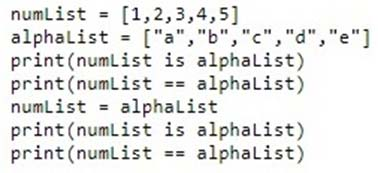Use the drop-down menus to select the answer choice that answers each question based on the information presented in the code segment.
Hot Area: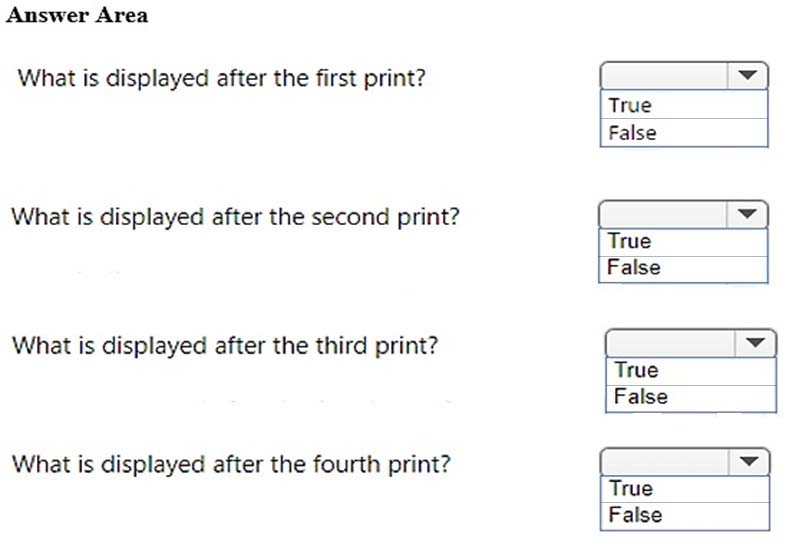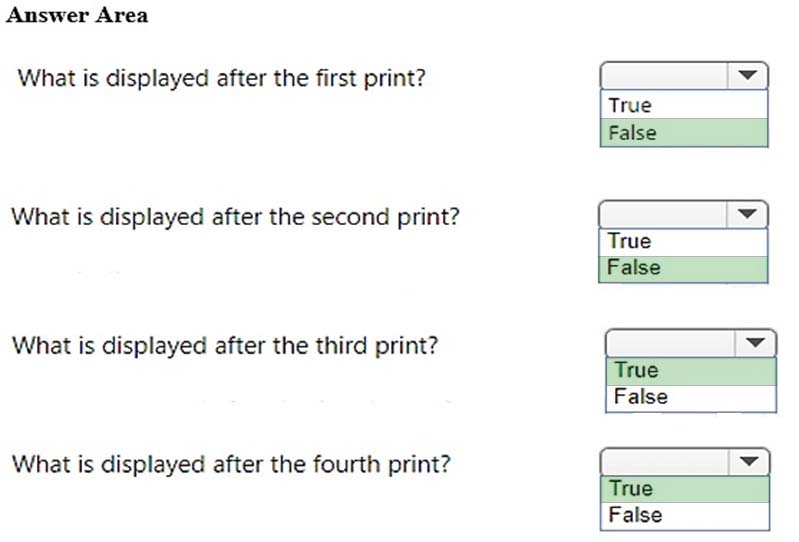References: https://www.w3resource.com/python/python-list.php

DRAG DROP -
You are writing a Python program to perform arithmetic operations.
You create the following code: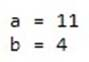What is the result of each arithmetic expression? To answer, drag the appropriate expression from the column on the left to its result on the right. Each expression may be used once, more than once, or not at all.
Select and Place: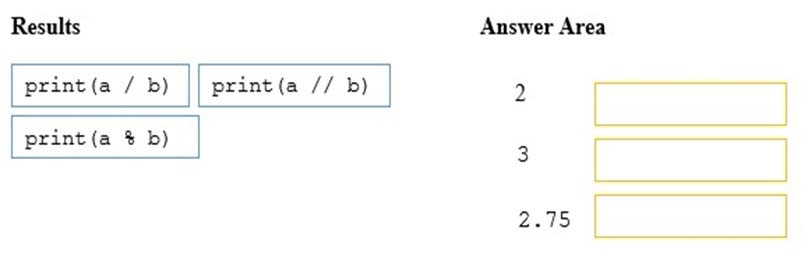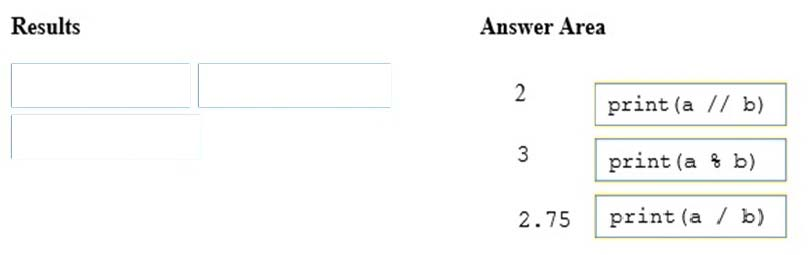References: https://www.w3resource.com/python/python-operators.php

DRAG DROP -
You are writing a Python program that evaluates an arithmetic formula.
The formula is described as b equals a multiplied by negative one, then raised to the second power, where a is the value that will be input and b is the result.
You create the following code segment. Line numbers are included for reference only.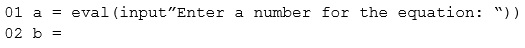You need to ensure that the result is correct.
How should you complete the code on line 02? To answer, drag the appropriate code segment to the correct location. Each code segment may be used once, more than once, or not at all. You may need to drag the split bar between panes or scroll to view content.
NOTE: Each correct selection is worth one point.
Select and Place: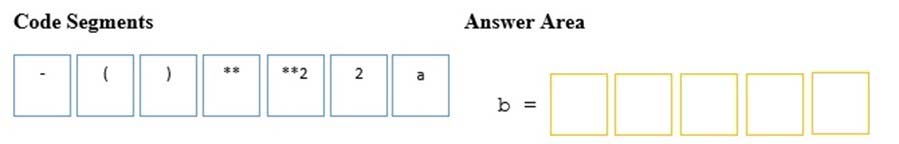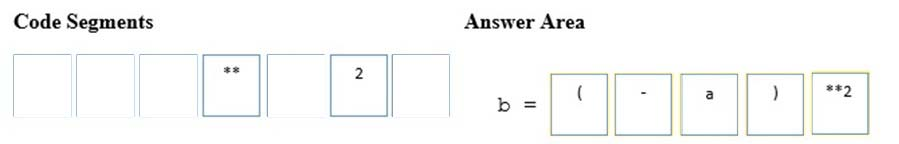Evaluate the following Python arithmetic expression: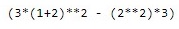What is the result?

• A. 3
• B. 13
• C. 15
• D. 69

References: http://www.mathcs.emory.edu/~valerie/courses/fall10/155/resources/op_precedence.html

You develop a Python application for your company.
A list named employees contains 200 employee names, the last five being company management. You need to slice the list to display all employees excluding management.
Which two code segments should you use? Each correct answer presents a complete solution. (Choose two.)

• A. employees [1:-4]
• B. employees [:-5]
• C. employees [1:-5]
• D. employees [0:-4]
• E. employees [0:-5]

References: https://www.w3resource.com/python/python-list.php#slice

HOTSPOT -
You are an intern for ABC electric cars company. You must create a function that calculates the average velocity of their vehicles on a 1320 foot (1/4 mile) track.
The output must be as precise as possible.
How should you complete the code? To answer, select the appropriate code segments in the answer area.
Hot Area: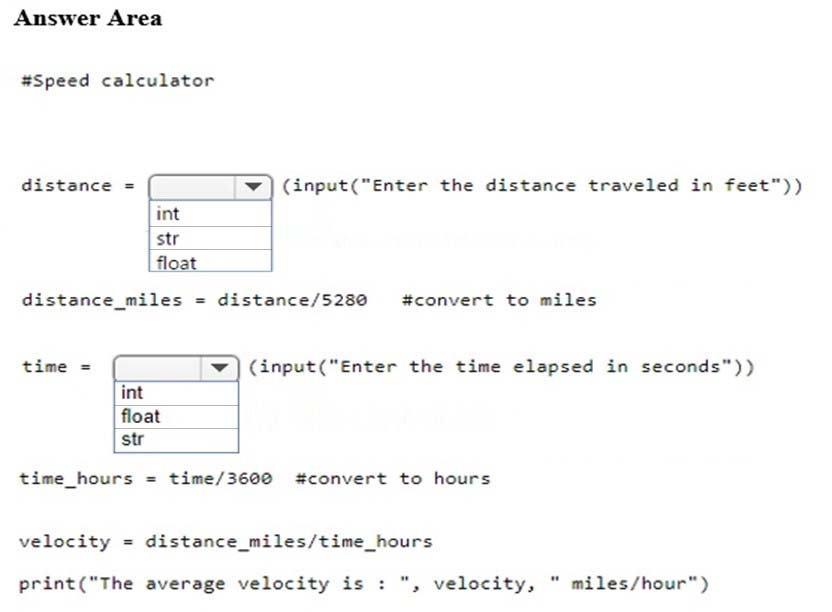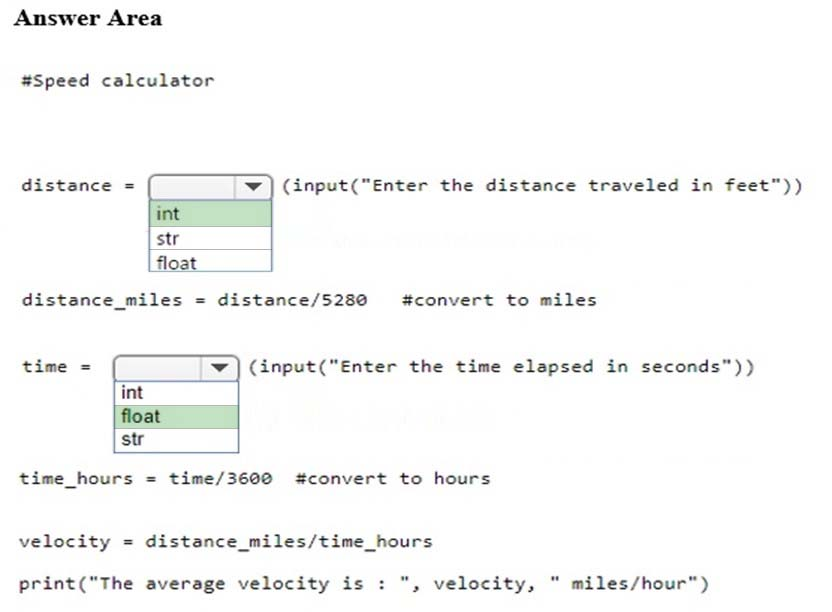References: https://www.w3resource.com/python/python-data-type.php

You are creating a function that manipulates a number. The function has the following requirements:
-> A float is passed into the function
-> The function must take the absolute value of the float
-> Any decimal points after the integer must be removed
Which two math functions should you use? Each correct answer is part of the solution. (Choose two.)

• A. math.fmod(x)
• B. math.frexp(x)
• C. math.floor(x)
• D. math.ceil(x)
• E. math.fabs(x)

Explanation:
C: math.floor(x) returns the largest integer less than or equal to x.
E: math.fabs(x) returns the absolute value of x.
A: math.fmod() takes two variables
B: math.frexp(x) returns the mantissa and exponent of x as the pair (m, e). m is a float and e is an integer
D: math.ceil(x) returns the smallest integer greater than or equal to x
References:
https://docs.python.org/2/library/math.html#number-theoretic-and-representation-functions https://docs.python.org/3/library/math.html

You are writing an application that uses the sqrt function. The program must reference the function using the name squareRoot.
You need to import the function.
Which code segment should you use?

• A. import math.sqrt as squareRoot
• B. import sqrt from math as squareRoot
• C. from math import sqrt as squareRoot
• D. from math.sqrt as squareRoot

References: https://infohost.nmt.edu/tcc/help/pubs/python/web/import-statement.html

You are writing code that generates a random integer with a minimum value of 5 and a maximum value of 11.
Which two functions should you use? Each correct answer presents a complete solution. (Choose two.)

• A. random.randint(5, 12)
• B. random.randint(5, 11)
• C. random.randrange(5, 12, 1)
• D. random.randrange(5, 11, 1)

References: https://docs.python.org/3/library/random.html#

DRAG DROP -
You are writing a function that works with files.
You need to ensure that the function returns None if the file does not exist. If the file does exist, the function must return the first line.
You write the following code: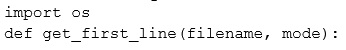In which order should you arrange the code segments to complete the function? To answer, move all code segments from the list of code segments to the answer area and arrange them in the correct order.
Select and Place: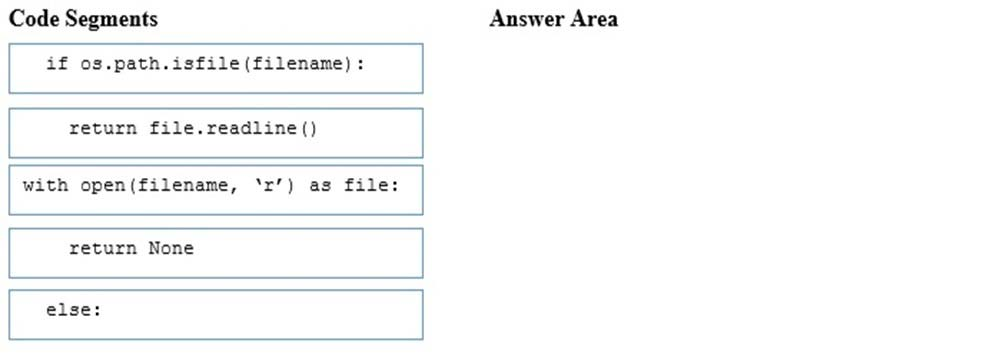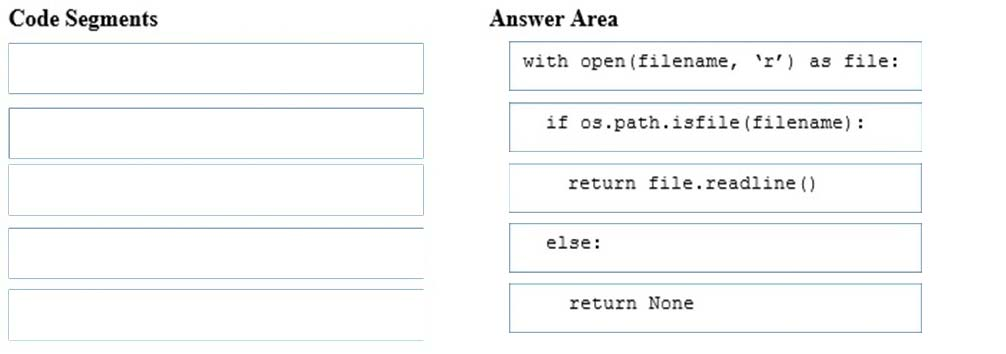Explanation:
References: http://effbot.org/zone/python-with-statement.htm

You are writing a Python program to automate inventory. Your first task is to read a file of inventory transactions. The file contains sales from the previous day, including the item id, price, and quantity.
The following shows a sample of data from the file: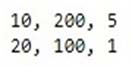The code must meet the following requirements:
-> Each line of the file must be read and printed
-> If a blank line is encountered, it must be ignored
-> When all lines have been read, the file must be closed
You create the following code. Line numbers are included for reference only.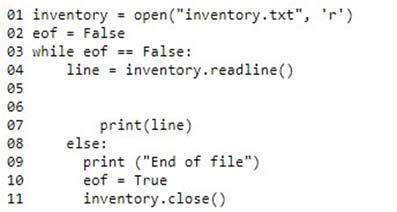Which code should you write for line 05 and line 06?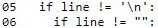A.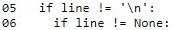B.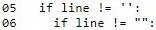C.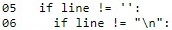D.

You develop a Python application for your company.
You need to accept input from the user and print that information to the user screen.
You have started with the following code. Line numbers are included for reference only.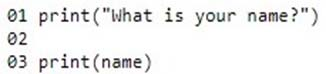Which code should you write at line 02?

• A. name = input
• B. input("name")
• C. input(name)
• D. name = input()

You develop a Python application for your school.
You need to read and write data to a text file. If the file does not exist, it must be created. If the file has content, the content must be removed.
Which code should you use?

• A. open("local_data", "r")
• B. open("local_data", "r+")
• C. open("local_data", "w+")
• D. open("local_data", "w")

Explanation:
Modes 'r+', 'w+' and 'a+' open the file for updating (reading and writing). Mode 'w+' truncates the file.
References:
https://docs.python.org/2/library/functions.html
https://pythontips.com/2014/01/15/the-open-function-explained/

Page:    1 / 3
Exam contains 43 questions